# Algebra 1 : Decimals and Percentage

## Example Questions

1 2 3 4 5 6 7 8 9 11 Next →

### Example Question #101 : Decimals And Percentage

Convert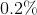to a decimal rounded to one decimal place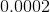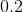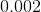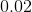Explanation:

To convert a percentage to a decimal, simply take the number and divide it by 100.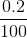### Example Question #57 : How To Find Decimal Equivalent To A Percentage

Convert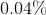to a decimal.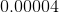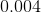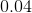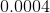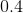Explanation:

Regardless if the percentage has a decimal in it or not, to convert a percent to a decimal take the percentage and divide it by 100.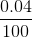### Example Question #102 : Decimals And Percentage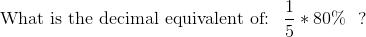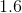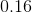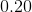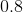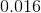Explanation:

First multiple the percentage by the fraction: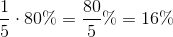Then divide the percentage value by 100 to get the decimal equivalent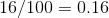### Example Question #101 : Decimals And Percentage

Convert the decimal to a percent:

0.55

50%

5%

0.55%

55%

5.5%

55%

Explanation:

To convert the decimal to a percent, move the decimal to the right two places, or multiply it by 100.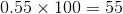1 2 3 4 5 6 7 8 9 11 Next →

### All Algebra 1 Resources